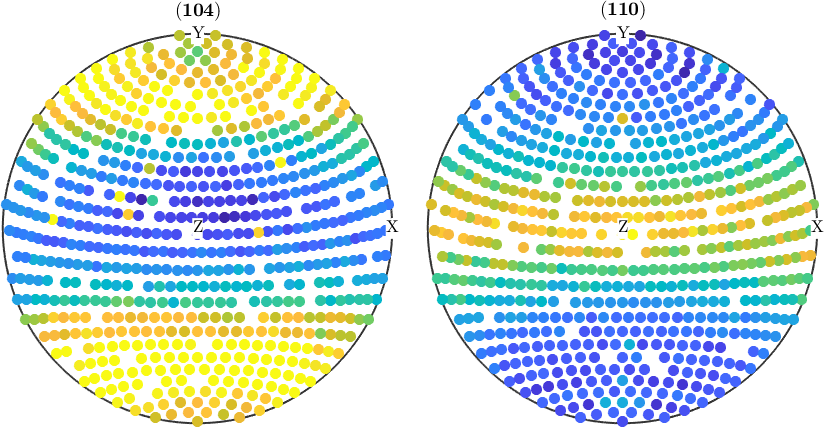Data Correction edit page

In this section we discuss how to manipulate pole figure data, e.g., to correct for measurement errors. To illustrate lets start by importing some sample XRD data.## Splitting and Reordering of Pole Figures

As we can see the first and the third pole figure complete pole figures and the second and the fourth pole figures contain some values for background correction. Let us, therefore, split the pole figures into these two groups.

Actually, it is possible to work with pole figures as with simple numbers. E.g. it is possible to add / subtract pole figures. A superposition of the first and the third pole figures can be written as

## Correct pole figure data

In order to correct pole figures for background radiation and defocusing one can use the command correct. In our case the syntax is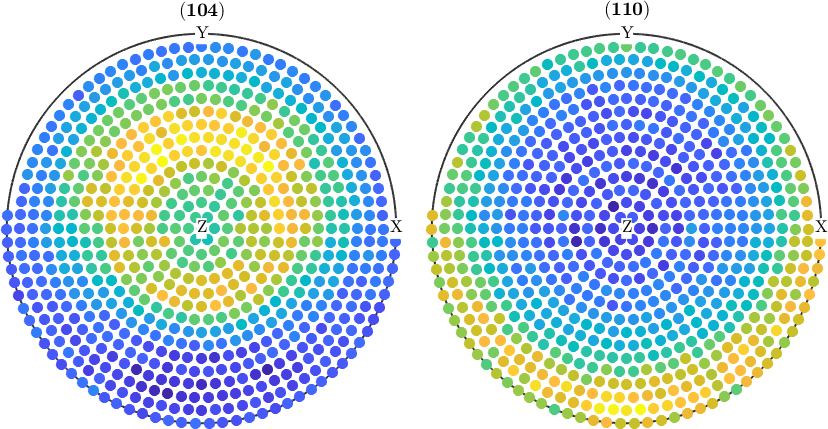## Normalize pole figures

Sometimes people want to have normalized pole figures. In the case of complete pole figures, this can be simply archived using the command normalize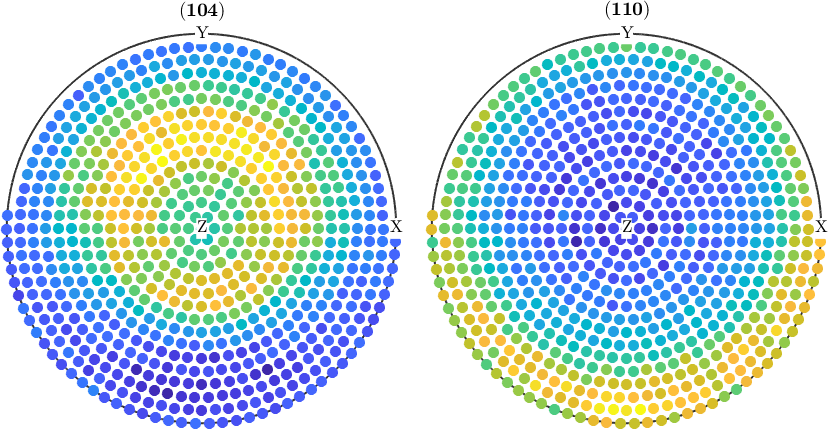However, in the case of incomplete pole figures, it is well known, that the normalization can only by computed from an ODF. Therefore, one has to proceed as follows: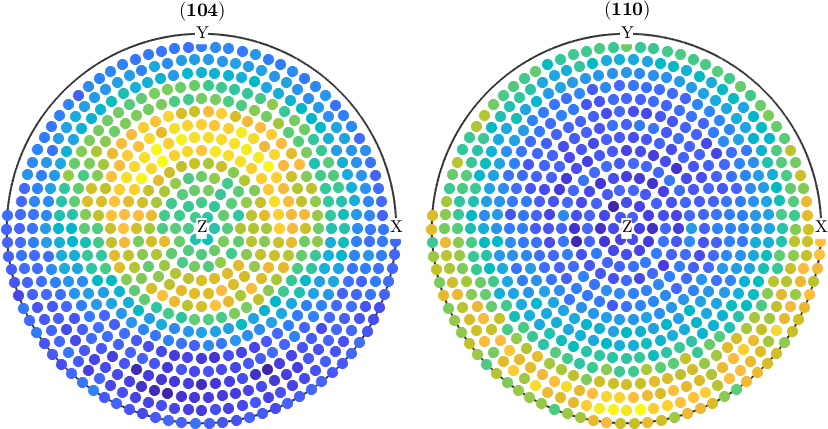## Modify certain pole figure values

As pole figures are usually experimental data they may contain outliers. In order to remove outliers from pole figure data one can use the function isOutlier. Here a simple example: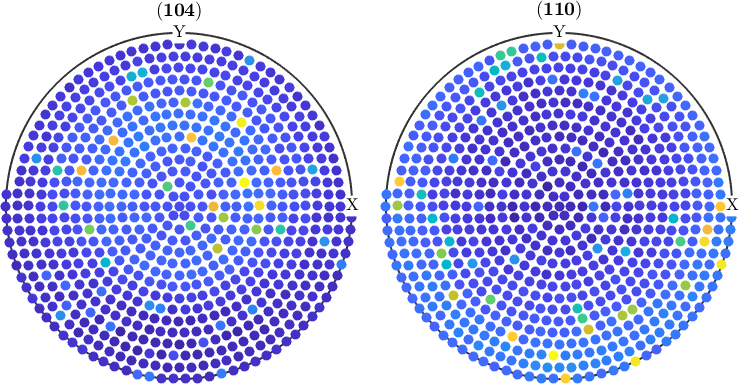check for outliers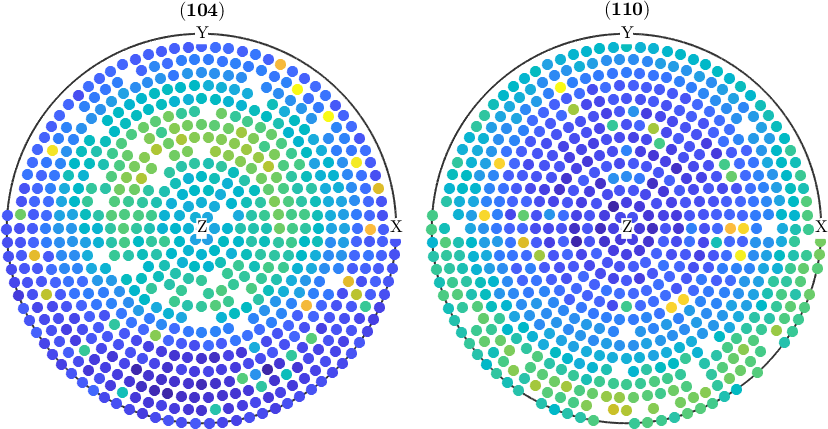Sometimes applying the above correction is not suffcient. Then it can help to repeat the outlier detection ones again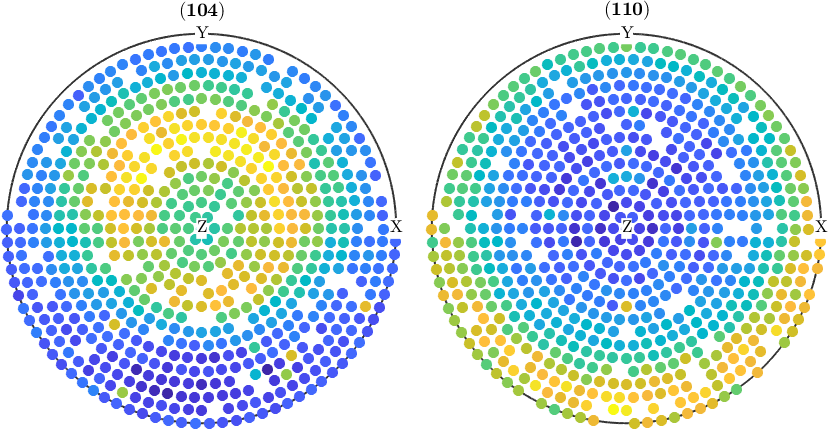## Remove certain measurements from the data

In the same way, as we removed the outlier one can manipulate and delete pole figure data by any criteria. Lets, e.g. cap all values that are larger than 500.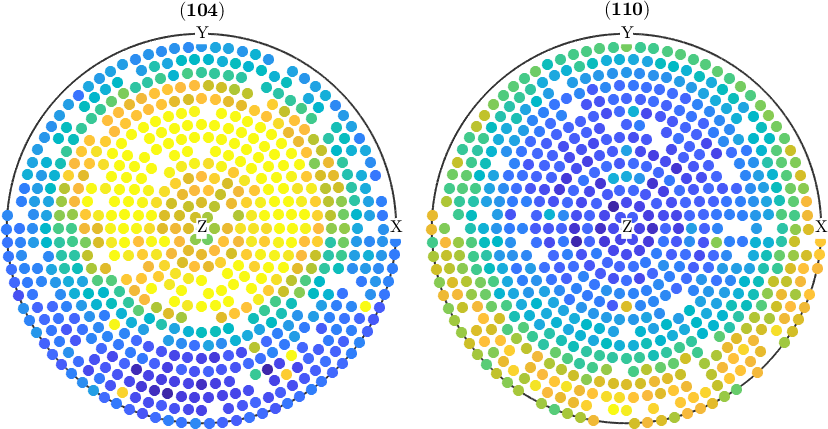## Rotate pole figures

Sometimes it is necessary to rotate the pole figures. In order to do this with MTEX one has first to define a rotation, e.e. by

Second, the command rotate can be used to rotate the pole figure data.